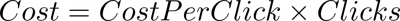Features

# Cost Per Click Calculator

Cost per click is a key digital marketing metric that is often associated with Google Search Ads or paid search advertising campaigns.

In digital marketing, the cost per click (CPC) represents the cost of each click on a given ad has cost. CPC is often associated closely with pay-per-click in search, while it is used alongside CTR and CPM for display advertising campaigns.

## Cost Per Click Formula

### Calculate CPC

Cost Per Click (CPC) is calculated by dividing the total cost by the total number of clicks.### Calculate Clicks

To calculate clicks from the cost per click and the total cost, divide the total cost by the cost per click. It is important to remember it is not possible to have a portion of a click, and any rounding in the cost per click used in this calculation may result in the number of clicks not being round.### Calculate Cost

To calculate the total cost from the cost per click and the total click, multiply the total clicks by the cost per click. Remember that if the cost per click has been rounded this could result in the total cost being incorrect.